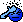# Development PHP Working with seconds...

Discussion in 'Software' started by nry, 24 May 2009.

1. ### nryMember

Joined:
1 Nov 2006
Posts:
513
4
Basically got a variable \$seconds, which holds a number of seconds up to 2 months

Making some code to calculate the number of sec, mins, hours, days, weeks and months are in this variable

So far I have this:

PHP:
```         \$days = intval(intval(\$seconds) / 86400);         //Calculate the hours         \$hours = intval(intval(\$seconds / 3600), 3600);         //Calculate the miniutes         \$minutes = bcmod((intval(\$seconds) / 60),60);         //Calculate the seconds         \$seconds = bcmod(intval(\$seconds),60); ```
Just the hours don't work.

Not got past days yet either.

Any help would be brilliant2. ### FirehedWhy not? I own a domain to match.

Joined:
15 Feb 2004
Posts:
12,574
16
Try \$hours = floor(\$seconds/3600); It looks like you're converting a valid value into base 3600, which is pretty much impossible (we don't have sufficient character sets to even represent that) and in any case will not bring the desired result.

3. ### thehippozNew Member

Joined:
19 Dec 2008
Posts:
5,780
174
Code:
```\$hourdiff = "-3"; // difference in hours between the web servers time and your time
\$disp = date("l, d F Y h:i a",time() + (\$hourdiff * 3600));
echo \$disp;
```
you can use something simple like this if you wanted to display the time and date.. output would look like this

Sunday, 24 May 2009 06:31 pm

* just re-read.. this is more along what you wanted

Code:
```\$s = 200000; // number of seconds set

\$d = intval(\$s/86400);

\$h = intval(\$s/3600);

\$m = intval(\$s/60);

echo \$d."d ". \$h ."h ". \$m ."m ". \$s."s";```
output for 20,000 seconds

2d 55h 3333m 200000s

Last edited: 25 May 2009
4. ### hitman012Active Member

Joined:
6 May 2005
Posts:
4,877
19
That would output the number of seconds converted to days, then converted to hours, then minutes, then seconds. He wants to convert it as a whole.

I haven't tested this, but you should be able to use the mod operator to find the remaining number of seconds after dividing to get days, hours etc:

PHP:
```  // Divide by seconds in a week\$weeks = floor(\$totalSeconds / 604800);// Get remainder after week division, divide by seconds in a day\$days = floor((\$totalSeconds % 604800) / 86400); // Get remainder after day division, divide by seconds in an hour\$hours = floor((\$totalSeconds % 86400) / 3600); // Get remainder after hour division, divide by seconds in a minute\$minutes = floor((\$totalSeconds % 3600) / 60); // Get remainder after division by minutes\$seconds = \$totalSeconds % 60;  echo "\$weeks weeks, \$days days, \$hours hours, \$minutes minutes and \$seconds seconds."; ```
Should be easy to extend to months, years etc if you want.

Edit: tested, works fine.

Last edited: 25 May 2009
5. ### thehippozNew Member

Joined:
19 Dec 2008
Posts:
5,780
174thanks hit.. that'll work- I was thinking- oh nm lol that's what he wants

6. ### BlackWhizzNew Member

Joined:
17 Jul 2008
Posts:
383
1
Why? PHP found out timestamps for this:

Code:
```\$timestamp = 20000000;
echo date('\D\a\y: d \M\o\n\t\h m \Y\e\a\r Y H:i:s', \$timestamp);
```

7. ### thehippozNew Member

Joined:
19 Dec 2008
Posts:
5,780
174
yeah was thinking that too whizz when first read it.. but it looks like he needs the values put into strings for whatever he's working on

Joined:
17 Jul 2008
Posts:
383